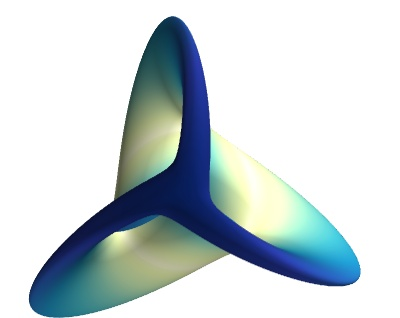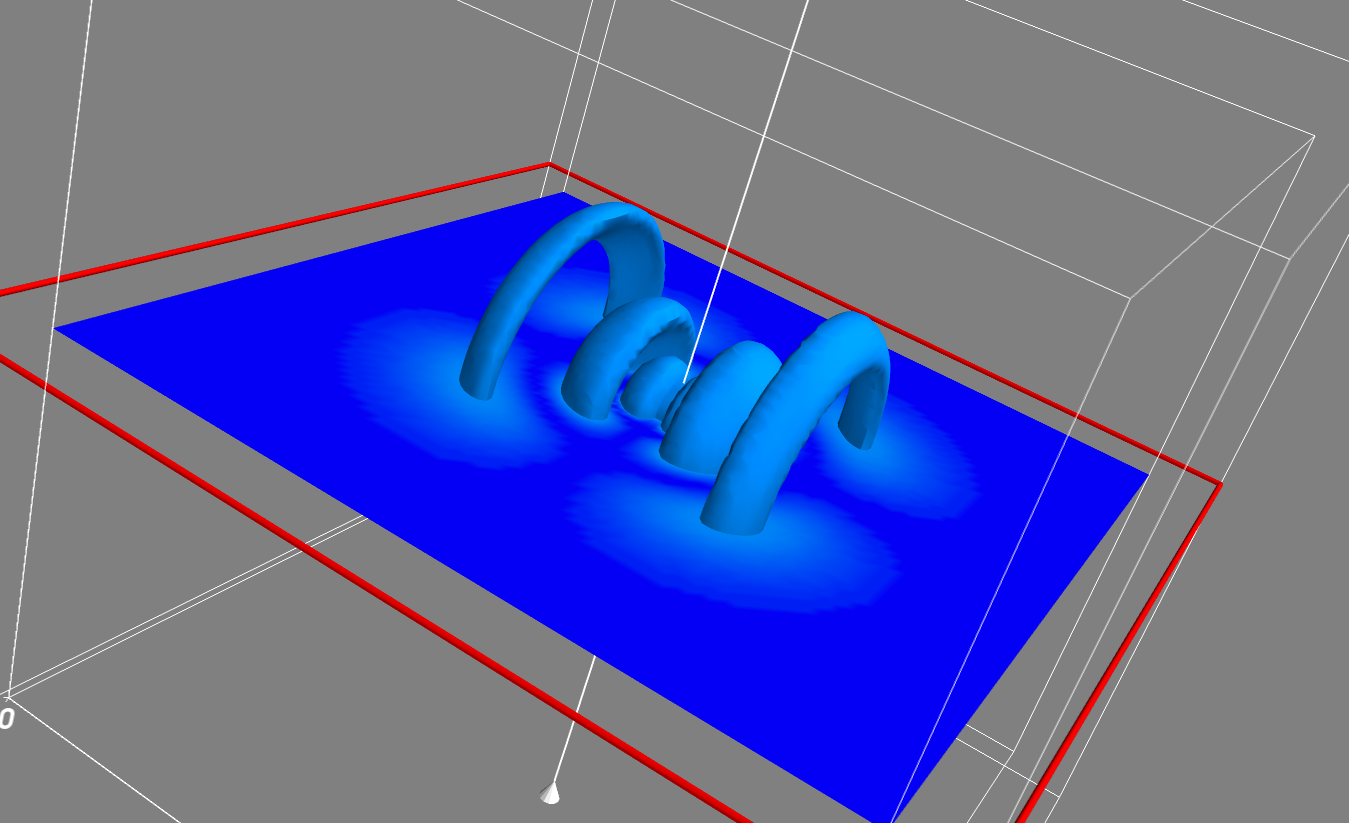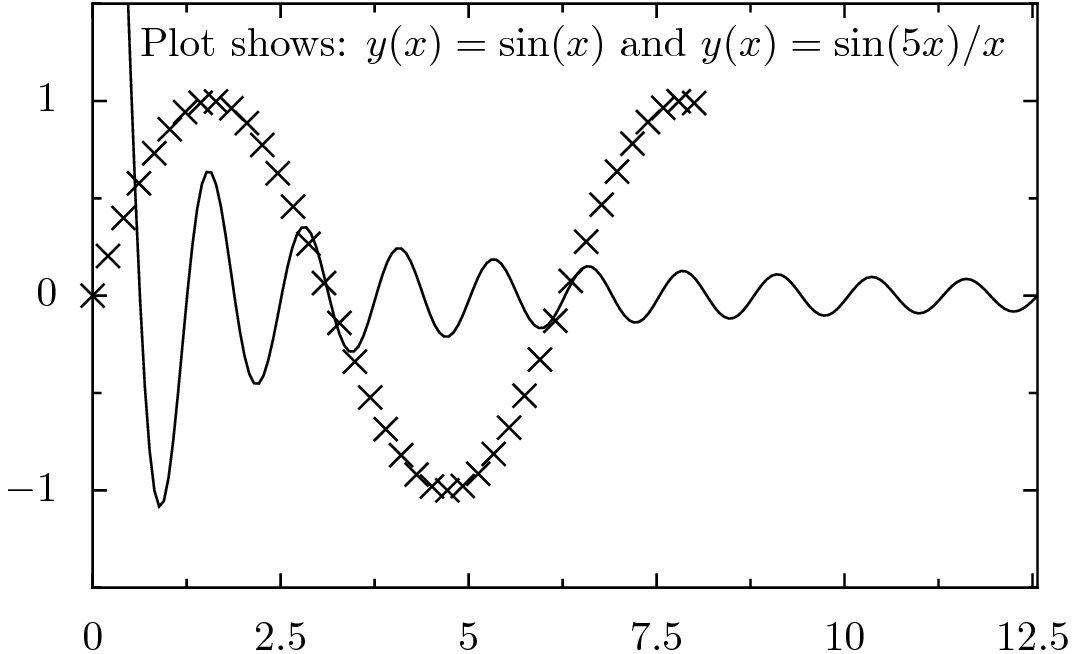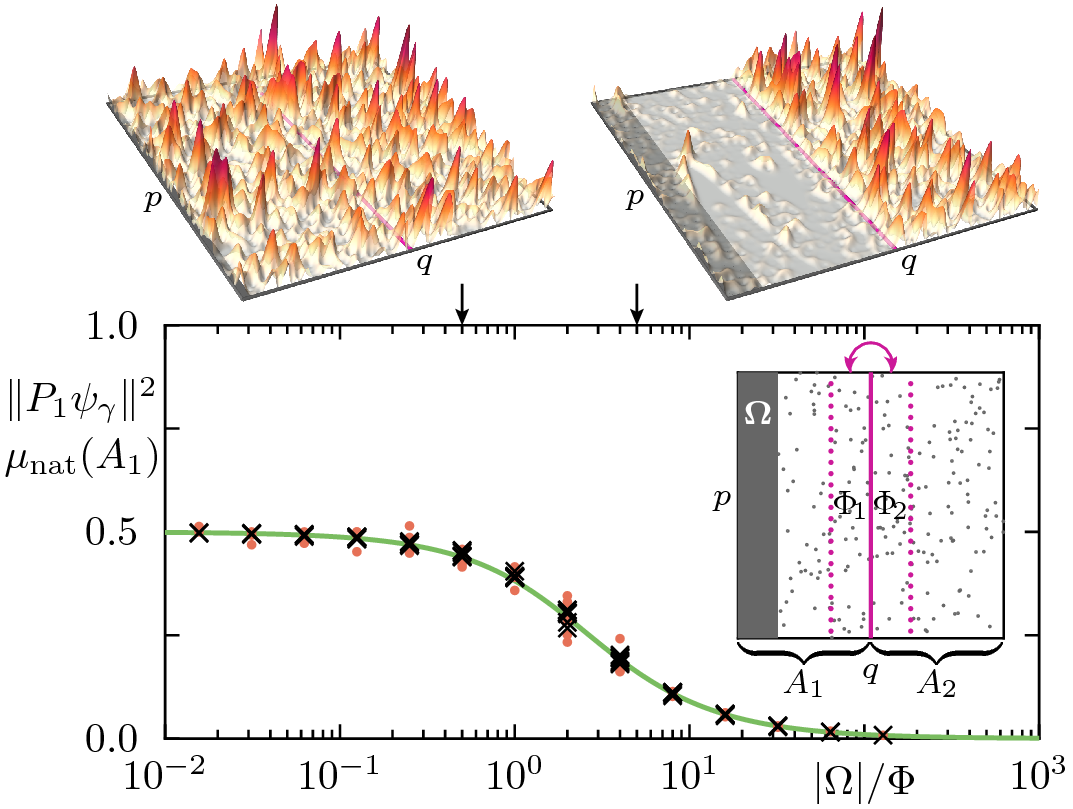## Tools for Scientific Computation

.

Python rocks

.

Open source is important...

.

https://wwwpub.zih.tu-dresden.de/~baecker/misc/py4science

.

Talk at IIT Madras, Arnd Bäcker, 28th February 2017

• Choosing the right tools

• Numerical computations

• Plotting:

• 2D graphics

• Interactive plots (react on mouse clicks)

• 3D visualizations

• Publication quality plots

• Symbolic computations

• Application writing

• Tools for collaborative work

## Choosing the right tools

Basic decision: Closed source vs. Open Source

Eg.: matlab/mathematica?

• (+) well established, well tested

• (+) good documentation

• (+) many toolboxes

• (-) expensive

• (-) complicated license set-up (does it work in the plane?)

• (-) availability at other universities?

• (-) running on cluster of CPUs?

• (-) ...

Good alternative: python + numpy + scipy + matplotlib + ...

## Numerical computations

Classical languages

• Fortran

• C/C++

Time-consuming to write, runs fast.

High-level languages

• Python

• Julia ...

Fast(er) to write, may run slower than required.

For speed: if necessary: use Cython/embed C/C++ code.

Important Coding time (your time) vs. running time.

## Python - some simple examples

Interactive usage with ipython:

import numpy as np
x = np.linspace(0.0, 1.0, 10)
y = np.sin(x)
print(x)
print(y)

Array operations:

z = x + 5 * y

## Python - Useful packages

• Python: full programming language, large standard library

• numpy: array operations

• scipy: linear algebra, special functions, ....

• matplotlib: 2D (and 3D) plotting

• mayavi: 3D visualization (docu)

## Numerical computations I

Matrix diagonalization:

import numpy as np
mat = np.random.rand(10, 10)
np.linalg.eigvals(mat)
np.linalg.eig(mat)

Singular value decomposition:

np.linalg.svd(mat)

And (among others):

• Solve linear system of equations

• QR decomposition

• Cholesky decomposition

For even more, see scipy.linalg.

## Numerical computations II

Fast Fourier transform:

import numpy as np
from scipy.fftpack import fft
x = np.linspace(0.0, 2.0*np.pi, 100, endpoint=False)
y = np.sin(x)
fft_y = fft(y)
print(fft_y)

Much more, see:

import scipy
help(scipy)

## Plotting I: 2D graphics, matplotlib

http://matplotlib.org/users/screenshots.html

Example:

ipython --matplotlib

from matplotlib import pyplot as plt

x = np.linspace(0.0, 10.0, 100)
plt.plot(x, np.sin(x), lw=4)

Remarks

• Excellent quality for bitmapped output (.jpg, .png).

• Good for quick plots

• Interactive plots

• Output is not of publication quality (IMHO)

## Plotting II: 2D graphics, mouse interaction

Code structure:

plt.subplot(111, aspect=1.0)
plt.axis([-1, 1, -1, 1])
plt.xlabel("x")
plt.ylabel("y")

[...]
plt.plot(x_pkt, y_pkt)      # plot something

plt.connect('button_press_event', function_called_on_click)

plt.show()

Examples: spirale.py, kicked_rotor.py

## Plotting III: 3D graphics: mayavi

import numpy as np
from mayavi import mlab

def f(x, y):
return np.sin(x+y) +  np.sin(2*x - y) + np.cos(3*x+4*y)

def show_surface():
"""Show surface on regularly spaced coordinates."""
x, y = np.mgrid[-7.:7.05:0.1, -5.:5.05:0.05]
surface = mlab.surf(x, y, f)
mlab.outline()
mlab.axes()
mlab.show()

show_surface()

## Plotting III: 3D graphics: mayavi## Plotting III: 3D graphics: mayavi## Plotting III: 3D graphics: mayavi

Generate 3D data set for probability density.

Visualize:

mayavi2 -d 5_2_1.vtk -m IsoSurface -m ScalarCutPlane -m Axes -m Outline## Plotting IV: publication quality plots: pyx

Webpage: http://pyx.sourceforge.net/

import numpy as np
import pyx

x = np.linspace(0.0, 8.0, 40)
y = np.sin(x)

xaxis = pyx.graph.axis.lin(min=0, max=4*np.pi)
yaxis = pyx.graph.axis.lin(min=-1.5, max=1.5)
pgr = pyx.graph.graphxy(width=8, x=xaxis, y=yaxis)
pgr.plot(pyx.graph.data.values(x=x, y=y))
pgr.plot(pyx.graph.data.function("y(x)=sin(5*x)/x", points=200))
pgr.text(0.4, 4.5, r"Plot shows: $y(x) = \sin(x)$ and $y(x)=\sin(5x)/x$")
pgr.writePDFfile("pyx_example")

## Plotting IV: publication quality plots: pyx

Webpage: http://pyx.sourceforge.net/

pyx_example.py - result## Plotting IV: publication quality plots: pyxFig. 1 from: M. J. Körber, A. B. and R. Ketzmerick,

Localization of Chaotic Resonance States due to a Partial Transport Barrier,

PRL 115 (2015) 254101

## Jupyter notebooks

http://jupyter.org/

Notebook for python code (and many other languages):

jupyter notebook

Point your browser to http://localhost:8888/.

Example:

%matplotlib inline
import matplotlib.pyplot as plt
import numpy as np

x = np.linspace(0.0, 10.0, 10)
y = np.sin(x)/x

plt.figure()
plt.plot(x, y, 'r')
plt.xlabel('x')
plt.ylabel('y')
plt.show()

Gallery of Notebooks

## Symbolic computations - SymPy

http://www.sympy.org, some examples: sympy notebook)

For example:

from sympy import *
init_printing()

x, y, z = symbols('x y z')
a = x**2 - 1
a
a.subs(x, y+1)

a = (x**3-y**3)/(x**2-y**2)
cancel(a)

trigsimp(2*sin(x)**2+3*cos(x)**2)

%matplotlib inline
plot(sin(x)/x, (x, -10, 10))

## Application writing

Trait objects can be modified by a GUI, traits_ui_example.py:

from traits.api import HasTraits, Str, Range

class Person(HasTraits):
first_name = Str("John")
last_name = Str("Cleese")
height = Range(0,3.0,1.80)

mr_x = Person()
mr_x.configure_traits(kind="modal")

Examples

• iterator

• explorator

## Collaborative working I: Exchanging files

Instead of dropbox, use

owncloud/nextcloud for:

• file exchange

• (shared) calenders

• note taking (shared documents)

## Collaborative working II: git

Version control using git for: code, notes, publications,...

Local usage:

git init
emacs first_file.txt
git commit -m "first file added."

Status, History, differences:

git status
git log
git diff  VersionHashCode

Collaborative usage: Server needed:

• gitlab installation of the computing centre (ask them!)

• overleaf.com for shared LaTeX documents

## Summary

• Have a look at: python + numpy, scipy, matplotlib, ...

• 3D visualization: mayavi
• For publication quality plots: pyx
• Tools for collaborative work: owncloud/nextcloud, git,...